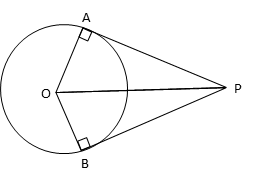# Past Year CBSE Board Paper

2017 Class 10 Math - Set 3 - Q26. Circles & Tangents

#### Video ExplanationPA and PB are tangents drawn to circle with center ‘O’ from external point P.

The angle between the radius and the tangents where the tangents meet the circle is a right angle.
So, ∠OAP = ∠OBP = 90°

OP is a common hypotenuse of right triangles OAP and OBP.

OB and OA are radii to the circle and are equal.

In right ΔOAP, PA = $\sqrt {{OP}^{2}-{OA}^{2}}$
In right ΔOBP, PB = $\sqrt {{OP}^{2}-{OB}^{2}}$

Because OA = OB, $\sqrt {{OP}^{2}-{OA}^{2}}$ = $\sqrt {{OP}^{2}-{OB}^{2}}$
Or PA = PB

.